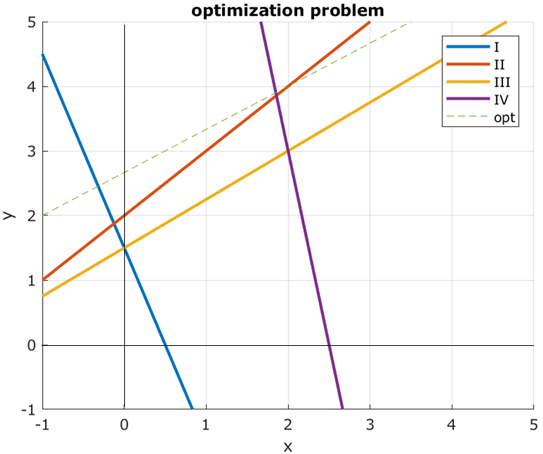# Constraint programming (Optimization)

One interesting and powerful albeit seldom used way of formulating a real-world problem in the computer is through constraint programming or integer programming.

What makes it difficult is finding variables that describe the problem abstractly in a way that they cover all properties you want to integrate. Through these variables complex real-life problems can be solved by maximizing or minimizing a cost function. Logical rules limiting our possibilities are formulated as inequations that demarcate the solutions space to a polyhedron in which we then optimize a cost function.

A biological example that can be tackled with constraint programming is the problem of finding the best 2D folding of a molecule like RNA. We want to maximize the number of hydrogen bonds on a given number of nucleotides without using any of them twice and without being sterically impossible.

##### Imagine that x and y are the concentrations of two molecules in the body that are coupled somehow. Find the polyhedron that represents all possible solutions on the defined variables.

blue

red

orange

purple

1.

y ≤ x + 2

2.

y ≤ –6*x + 15

3.

y ≥ – 3*x +1.5

4.

y ≤  ¾*x + 1.5

Like in school calculate the slope and the intercept which in these functions are easy to read directly from the plot. These functions together with the very commonly used additional functions x ≥ 0 and y ≥ 0 define the polyhedron and have to be written into a program. The cost function is optimized by following the rim of the polyhedron. Usually problems are formulated in matlab and solved with the package gurobi.

Like in school calculate the slope and the intercept which in these functions are easy to read directly from the plot. These functions together with the very commonly used additional constraints x ≥ 0 and y ≥ 0 define the polyhedron and have to be written into a linear program. The cost function can be optimized by walking along the edges of the polyhedron.

##### Find the points which maximize or minimize the optimization function f(x,y) = 2*x – 3*y. Imagine for example that you need certain amounts of molecules x and y for a chemical reaction and want to know how much is produced. Additionally identify the invalid point and a random point which satisfies the constraints.1.

minimum

Move the cost function down until you reach the point (2,3) with f(2,3)= –5.

2.

random possible

This point lies on the boundary of the polyhedron. Since the inequalities all contain an equality sign this point is valid, but the cost in this point is not as big or small as in the other marked points.

3.

maximum

Move the cost function down until you reach the point (2.5,0) with f(2.5,0)= 5.

4.

not valid

The inequality y ≤ ¾*x + 1.5 (orange) makes this point invalid.

You get feedback for each answer by clicking on the button.